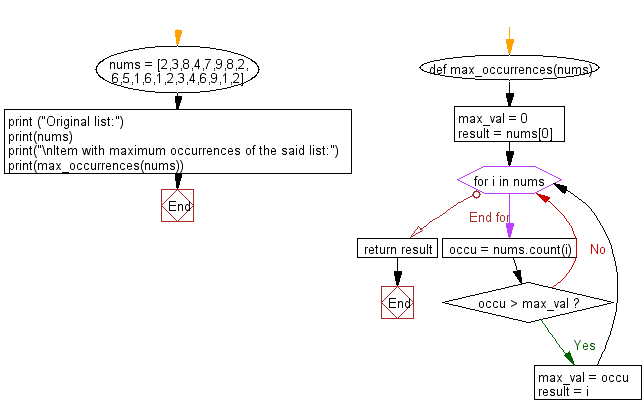﻿ Python: Find the item with maximum occurrences in a given list - w3resource# Python: Find the item with maximum occurrences in a given list

## Python List: Exercise - 110 with Solution

Write a Python program to find the item with maximum occurrences in a given list.

Sample Solution:

Python Code:

``````def max_occurrences(nums):
max_val = 0
result = nums
for i in nums:
occu = nums.count(i)
if occu > max_val:
max_val = occu
result = i
return result

nums = [2,3,8,4,7,9,8,2,6,5,1,6,1,2,3,4,6,9,1,2]
print ("Original list:")
print(nums)
print("\nItem with maximum occurrences of the said list:")
print(max_occurrences(nums))
```
```

Sample Output:

```Original list:
[2, 3, 8, 4, 7, 9, 8, 2, 6, 5, 1, 6, 1, 2, 3, 4, 6, 9, 1, 2]

Item with maximum occurrences of the said list:
2
```

Pictorial Presentation:Flowchart:## Visualize Python code execution:

The following tool visualize what the computer is doing step-by-step as it executes the said program:

Python Code Editor:

Have another way to solve this solution? Contribute your code (and comments) through Disqus.

What is the difficulty level of this exercise?

Test your Programming skills with w3resource's quiz.

﻿

## Python: Tips of the Day

Clamps num within the inclusive range specified by the boundary values x and y:

Example:

```def tips_clamp_num(num,x,y):
return max(min(num, max(x, y)), min(x, y))
print(tips_clamp_num(2, 4, 6))
print(tips_clamp_num(1, -1, -6))
```

Output:

```4
-1
```